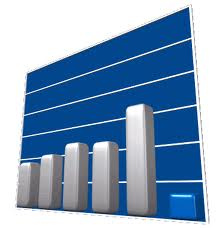Statistics is a mathematical science pertaining to the collection, analysis, interpretation or explanation, and presentation of data. Statisticians improve the quality of data with the design of experiments and survey sampling. Statistics also provides tools for prediction and forecasting using data and statistical models. Statistics is applicable to a wide variety of academic disciplines, including natural and social sciences, government, and business.

### Meant by statistics

Statistical methods can be used to summarize or describe a collection of  statistical methods statistics data; this is called descriptive statistics. This is useful in research, when communicating the results of experiments. In addition, patterns in the data may be modeled in a way that accounts for randomness and uncertainty in the observations, and are then used to draw inferences about the process or population being studied; this is called inferential statistics. Inference is a vital element of scientific advance, since it provides a prediction (based in data) for where a theory logically leads. To further prove the guiding theory, these predictions are tested as well, as part of the scientific method. If the inference holds true, then the descriptive statistics of the new data increase the soundness of that hypothesis. Descriptive statistics and inferential statistics together comprise applied statistics.

The word statistics can either be singular or plural. In its singular form, statistics refers to the mathematical science discussed in this article. In its plural form, statistics is the plural of the word statistic, which refers to a quantity (such as a mean) calculated from a set of data.